International
Tables for
Crystallography
Volume C
Mathematical, physical and chemical tables
Edited by E. Prince

International Tables for Crystallography (2006). Vol. C, ch. 8.4, pp. 705-706

## Section 8.4.4. Influence of individual data points

E. Princea and C. H. Spiegelmanb

aNIST Center for Neutron Research, National Institute of Standards and Technology, Gaithersburg, MD 20899, USA, and bDepartment of Statistics, Texas A&M University, College Station, TX 77843, USA

### 8.4.4. Influence of individual data points

| top | pdf |

When the method of least squares, or any variant of it, is used to refine a crystal structure, it is implicitly assumed that a model with adjustable parameters makes an unbiased prediction of the experimental observations for some (a priori unknown) set of values of those parameters. The existence of any reflection whose observed intensity is inconsistent with this assumption, that is that it differs from the predicted value by an amount that cannot be reconciled with the precision of the measurement, must cause the model to be rejected, or at least modified. In making precise estimates of the values of the unknown parameters, however, different reflections do not all carry the same amount of information (Shoemaker, 1968; Prince & Nicholson, 1985). For an obvious example, consider a space-group systematic absence. Except for possible effects of multiple diffraction or twinning, any observed intensity at a position corresponding to a systematic absence is proof that the screw axis or glide plane is not present. If no intensity is observed for any such reflection, however, any parameter values that conform to the space group are equally acceptable. It is to be expected, on the other hand, that some intensities will be extremely sensitive to small changes in some parameter, and that careful measurement of those intensities will lead to correspondingly precise estimates of the parameter values. For the purpose of precise structure refinement, it is useful to be able to identify the influential reflections.

Consider a vector of observations, y, and a model M(x). The elements of y define an n-dimension space, and the model values, Mi(x), define a p-dimensional subspace within it. The least-squares solution [equation (8.1.2.7)],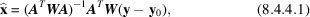is such that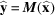is the closest point to y that corresponds to some possible value of x. In (8.4.4.1), W = V−1 is the inverse of the variance–covariance matrix for the joint p.d.f. of the elements of y, and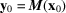is a point in the p-dimensional subspace close enough to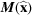so that the linear approximation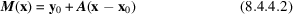[where] is a good one. Let R be the Cholesky factor of W, so that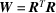, and let Z = RA,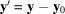, and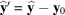. The least-squares estimate may then be written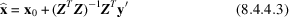and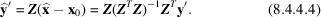Thus, the matrix P = Z(ZTZ)ZT, the projection matrix, is a linear relation between the observed data values and the corresponding calculated values. (Because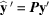, the matrix P is frequently referred to in the statistical literature as the hat matrix.) P2 = Z(ZTZ)− 1ZTZ(ZTZ)−1ZT = Z(ZTZ)−1ZT = P, so that P is idempotent. P is an n × n positive semidefinite matrix with rank p, and its eigenvalues are either 1 (p times) or 0 (np times). Its diagonal elements lie in the range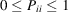, and the trace of P is p, so that the average value of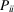is p/n. Furthermore,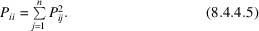A diagonal element of P is a measure of the influence that an observation has on its own calculated value. Ifis close to one, the model is forced to fit the ith data point, which puts a constraint on the value of the corresponding function of the parameters. A very small value of, because of (8.4.4.5), implies that all elements of the row must be small, and that observation has little influence on its own or any other calculated value. Because it is a measure of influence on the fit,is sometimes referred to as the leverage of the ith observation. Note that, because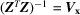, the variance–covariance matrix for the elements of,is the variance–covariance matrix for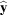, whose elements are functions of the elements of. A large value ofmeans thatis poorly defined by the elements of, which implies in turn that some elements ofmust be precisely defined by a precise measurement of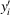.

It is apparent that, in a real experiment, there will be appreciable variation among observations in their leverage. It can be shown (Fedorov, 1972; Prince & Nicholson, 1985) that the observations with the greatest leverage also have the largest effect on the volume of the p-dimensional confidence region for the parameter estimates. Because this volume is a rather gross measure, however, it is useful to have a measure of the influence of individual observations on individual parameters. Let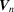be the variance–covariance matrix for a refinement including n observations, and let z be a row vector whose elements are zj =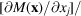σ for an additional observation.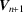, the variance–covariance matrix with the additional observation included, is, by definition,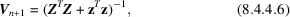which, in the linear approximation, can be shown to be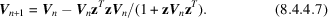The diagonal elements of the rank one matrix D = VnzTzVn/(1 + zVnzT) are therefore the amounts that the variances of the estimates of individual parameters will be reduced by inclusion of the additional observation.

This result depends on the elements of Z and z not changing significantly in the (presumably small) shift from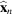to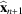. That this condition is satisfied may be verified by the following procedure. Find an approximation toby a line search along the line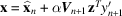, and then evaluate B, a quasi-Newton update such as the BFGS update (Subsection 8.1.4.3) at that point. If α = 1, and the gradient of the sum of squares vanishes, then the linear approximation is exact, and B is null. If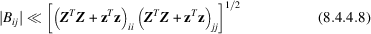for all i and j, then (8.4.4.7)can be expected to be an excellent approximation for a nonlinear model.

### References

Fedorov, V. V. (1972). Theory of optimal experiments, translated by W. J. Studden & E. M. Klimko. New York: Academic Press.
Prince, E. & Nicholson, W. L. (1985). Influence of individual reflections on the precision of parameter estimates in least squares refinement. Structure and statistics in crystallography, edited by A. J. C. Wilson, pp. 183–195. Guilderland, NY: Adenine Press.
Shoemaker, D. P. (1968). Optimization of counting time in computer controlled X-ray and neutron single-crystal diffractometry. Acta Cryst. A24, 136–142.